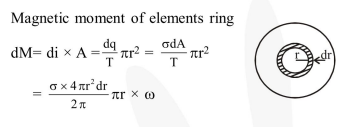Deepak Scored 45->99%ile with Bounce Back Crack Course. You can do it too!

# A thin circular disk of radius R is uniformly charged

Question:

A thin circular disk of radius $R$ is uniformly charged with density $\sigma>0$ per unit area. The disk rotates about its axis with a uniform angular speed $\omega$. The magnetic moment of the disk is :-

1. $2 \pi R^{4} \sigma \omega$

2. $\pi R^{4} \sigma \omega$

3. $\frac{\pi \mathrm{R}^{4}}{2} \sigma \omega$

4. $\frac{\pi R^{4}}{4} \sigma \omega$

Correct Option: , 4

Solution: